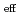JAEA develops a new nuclear data processing system FRENDY. We investigated all processing methods and we focused on the probability table generation using the ladder method which is adopted in the PURR module in NJOY. To improve the probability table generation, the more sophisticated method was introduced in the calculation methods of the Chi-Squared random numbers and the complex error function. We also investigated the appropriate ladder number. To investigate the impact of the difference of the complex error function calculation method, the Kvalues of the benchmark experiments with the probability tables by the both methods were compared. The calculation results indicated that the appropriate ladder number is 100 and the difference of the calculation methods of the Chi-Squared random numbers and the complex error function has no significant impact on the neutronics calculation.